# Mental Arithmetic – Multiplications

### Score

New Quiz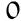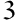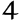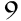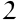Players

When we talk about multiplication, we’re talking about a fundamental arithmetic operation, often represented by the symbol ×. Simplified to the extreme, multiplication consists in combining equal groups to obtain a total. Take an elementary example: 2 × 3 is equivalent to adding the number 2, three times, to obtain 6.

The discovery of multiplication dates back to antiquity, when it was used to simplify calculations in commerce, agriculture and engineering. From the abacus to modern algorithms, multiplication has undergone a remarkable evolution, facilitating increasingly complex calculations.

Multiplication is not limited to the well-known decimal system. Take the binary system, essential in computing: here, multiplying means moving numbers around and adding them up, a different process but just as crucial to the operation of today’s technologies.

Product is the mathematical term for the result of multiplication. If we multiply 4 by 5, the product is 20. This concept goes beyond simple arithmetic and plays an essential role in fields such as algebra, geometry and many others. To illustrate our point, let’s take the area of a rectangle: simply multiply the length by the width to obtain the area, a concrete application of the concept.

Mastering rapid multiplication techniques is a real asset. Methods such as multiplying by jalousies or using the Pythagorean table provide fast, efficient results. Regular practice, for example with mental arithmetic games or dedicated applications, can improve speed and accuracy.

Case studies show that the ability to perform rapid multiplication is invaluable in many situations, from day-to-day budget management to solving more complex problems in science or technology. Practising these skills is therefore not just an intellectual exercise, but also a practical tool for everyday life.